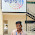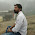# Hacker Rank solution For Day 20: Sorting

Problem:- Write a Hackerrank Solution For Day 20: Sorting or Hacker Rank Solution Program In C++ For " Day 20: Sorting " or Hackerrank 30 days of code Java Solution:Day 20: Sorting solution or Hackerrank solution for 30 Days of Code Challenges or Hackerrank 30 days of code Java Solution, Day 20: Sorting solution, or C/C++ Logic & Problem Solving: Day 20: Sorting or Hacker Rank Solution For Day 20: Sorting.

Check This:- Hacker rank solution for Strings, Classes, STL, Inheritance in C++.

Explanation:- This is a Simple case of sorting we have to sort the array and also count the total number of swap and minimum and maximum elements of an array this is an Advance Bubble Sort Example we also put the one special condition If array is already sorted than no need to run an array or for saving a time complexity if an array is already sorted than running time complexity is O(n). If an array is not sorted then we have to swap array elements and also count the swap how many swaps needed to sort an array. I strongly recommend checking some Sorting problem Like Bubble Sort, Selection Sort, Insertion Sort for full clear doubts.

Also Check:- Geeksforgeeks solution for School, Basic, Easy, Medium, Hard in C++.

Tip:- Copy the colored code or full code(According to Requirement ) and paste it into hacker rank editor.All solution provided here are in C++ (CPP) if any reader wants these solutions in C, and Java comments below or Email me with your query like " day n solution in C / C++ / Java. Check the end of the post solutions with the full explanation

Solution:-

#include <cmath>
#include <cstdio>
#include <vector>
#include <iostream>
#include <algorithm>
using namespace std;

int main()
{
/* Enter your code here. Read input from STDIN. Print output to STDOUT */
int i, j, n, temp, count=0, Swaps;
cin>>n;
int array[n];

for (i = 0; i <n; i++)
{
cin>>array[i];
}
for (i = n-1; i >0; i--)
{
Swaps = 0;
for (j = 0; j <i; j++)
{
if(array[j]>array[j+1])
{
temp=array[j];
array[j]=array[j+1];
array[j+1]=temp;
Swaps++;
count++;
}
}
if (Swaps == 0)
{
break;
}
}
cout<<"Array is sorted in "<<count<<" swaps.\n";
cout<<"First Element: "<<array<<endl;
cout<<"Last Element: "<<array[n-1]<<endl;
return 0;
}

Output:-

You May Also Like:-

1. Hackerrank Solution For Day 13: Abstract Classes

2. Hackerrank Solution For Day 14: Scope

3. Hackerrank Solution For Day 15: Linked List

4. Hackerrank Solution For Day 19: Interfaces

5. Hackerrank Solution For Day 21: Generics

6. Hackerrank Solution For Day 22: Binary Search Trees

7. Hackerrank Solution For Day 23: BST Level-Order Traversal

8. Hackerrank Solution For Day 24: More Linked Lists

9. Hackerrank Solution For Day 25: Running Time and Complexity

10. Hackerrank Solution For Day 26: Nested Logic

1.1.